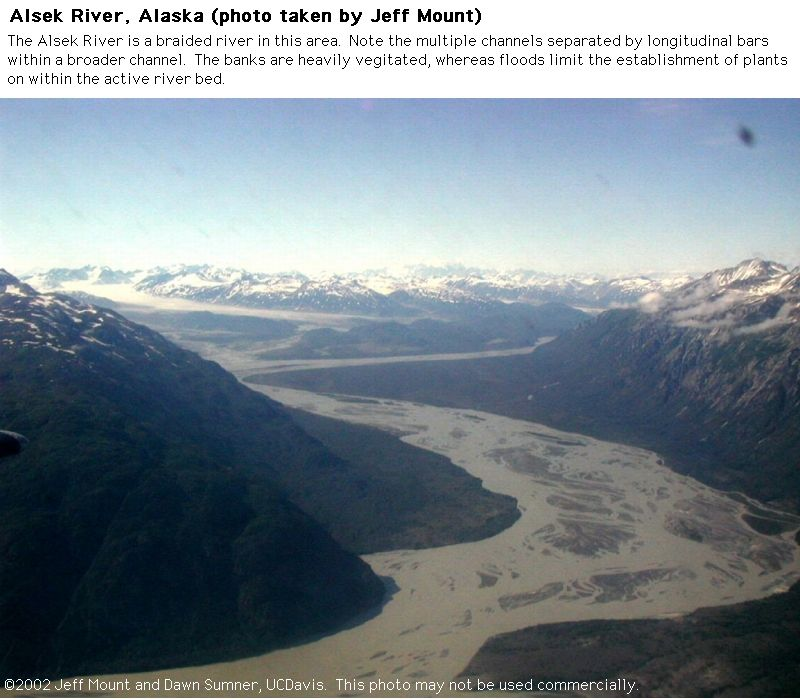# 6.1.5: Fluvial Environments (Chapter 9)

$$\newcommand{\vecs}{\overset { \rightharpoonup} {\mathbf{#1}} }$$ $$\newcommand{\vecd}{\overset{-\!-\!\rightharpoonup}{\vphantom{a}\smash {#1}}}$$$$\newcommand{\id}{\mathrm{id}}$$ $$\newcommand{\Span}{\mathrm{span}}$$ $$\newcommand{\kernel}{\mathrm{null}\,}$$ $$\newcommand{\range}{\mathrm{range}\,}$$ $$\newcommand{\RealPart}{\mathrm{Re}}$$ $$\newcommand{\ImaginaryPart}{\mathrm{Im}}$$ $$\newcommand{\Argument}{\mathrm{Arg}}$$ $$\newcommand{\norm}{\| #1 \|}$$ $$\newcommand{\inner}{\langle #1, #2 \rangle}$$ $$\newcommand{\Span}{\mathrm{span}}$$ $$\newcommand{\id}{\mathrm{id}}$$ $$\newcommand{\Span}{\mathrm{span}}$$ $$\newcommand{\kernel}{\mathrm{null}\,}$$ $$\newcommand{\range}{\mathrm{range}\,}$$ $$\newcommand{\RealPart}{\mathrm{Re}}$$ $$\newcommand{\ImaginaryPart}{\mathrm{Im}}$$ $$\newcommand{\Argument}{\mathrm{Arg}}$$ $$\newcommand{\norm}{\| #1 \|}$$ $$\newcommand{\inner}{\langle #1, #2 \rangle}$$ $$\newcommand{\Span}{\mathrm{span}}$$$$\newcommand{\AA}{\unicode[.8,0]{x212B}}$$Figure $$\PageIndex{1}$$: Fluvial Fan (Alaska)Figure $$\PageIndex{2}$$: Braided River (Alaska)Figure $$\PageIndex{3}$$: Braided River (Alaska)Figure $$\PageIndex{4}$$: Dunes migrating in a creekFigure $$\PageIndex{5}$$: Dunes migrating in a creekFigure $$\PageIndex{6}$$: Antidunes in a creekFigure $$\PageIndex{7}$$: Pebble scoursFigure $$\PageIndex{8}$$: Recent Alluvial Deposits (Terrace at Redrock State Park, CA)Figure $$\PageIndex{9}$$: Recent Alluvial Deposits (Terrace at Redrock State Park, CA)Figure $$\PageIndex{10}$$: Recent Alluvial Deposits (Death Valley, CA)Figure $$\PageIndex{11}$$: Channel Erosion Due to Changing Base Level/Flow Speeds (Death Valley, CA)Figure $$\PageIndex{12}$$: Ancient Trough Cross Stratification (two faces of the same rock)Figure $$\PageIndex{13}$$: Plan or Map View of Ancient Trough Cross StratificationFigure $$\PageIndex{14}$$: Ancient Trough Cross StratificationFigure $$\PageIndex{15}$$: Ancient Trough Cross StratificationFigure $$\PageIndex{16}$$: Fining Upward Fluvial CyclesFigure $$\PageIndex{17}$$: Small Fining Upward Fluvial CycleFigure $$\PageIndex{18}$$: Single set of cross strata in a planar bed​​​​​​​

Figure $$\PageIndex{19}$$: Recent Fluvial Deposits (Alsek River, Alaska)